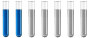Question about aluminium electrolysis (little complex)Author Message

Jr. MemberJoined: 08 Apr 2020
Posts: 5
Location: Brazil# Question about aluminium electrolysis (little complex)

First of all, I'm not a native english speaker, some terms maybe a little off

Basically, my professor asked how much liters of oxigen are produced based on an aluminium electrolysis in an industrial process, this is the equation:

2Al2O3 = 4Al + 3O2

This electrolysis was done at 900Celsius, using graphite electrodes, the anodic atracts the oxigen and form CO2 that is discarted, the catodic atracts pure liquid Aluminium that is trasnferred to another place, here is an image about it:
https://imgur.com/a/rz8gDwd (unfortunately I don't have it in english, it's in portuguese)

But before the oxigen calculation, he asked how much mass of Al is produced based on a 8 hours production using 135000amp, we rounded faraday's constant to 96500. The calculation went like this:

Each 4 moles of Al3+ need 12 moles of electrons;
m= 135000 x 28800 x 27 / 12 x 96500
m= ~1.04x1011 / 1158000
m= ~90652.8 grams of Aluminium = 90.6 Kilos of Aluminium

After we done this, he asked how many liters of oxigen are produced in this case at 2.5atm using the universal gas constant (0.082 L⋅atm⋅K^−1⋅mol^−1), but he also asked to do the calculation based on a 90.6 Kilos of aluminium production. My first thought was "well i'll just convert 90.6 kilos to moles, balance it and i'll have the results" I did it, but he said it's wrong, i don't know if I'm missing something, here's how I did:

First, converting to moles:
90600 / 27 = ~3355.5 Al moles
Balancing:
1677.75 Al2O3 = 3355.5 Al + 2516.625 O2
Calculation:
P x V = n x R x T
2.5 x V = 2516.625 x 0.082 x (900+273,15)
2.5V = ~242095.04
V = ~242095.04 / 2.5
V= ~96838.01 Liters of oxigen

Me and the boys did a debate and couldn't conclude if we're really wrong or the professor is. Asking for help on this question because he said the results and they are VERY far to mine and I don't even can thing how to reach them.Distinguished MemberJoined: 27 Sep 2017
Posts: 415
Location: Berlin, Germanym = I x t x 3600 x M/(z x 96500) m = 135000 A x 8 * 3600 s x 27 g/ mol/(3 x 96500 As) m = 362,61 kg There is no division by 12 because Faraday Law is calculated for 1 mol Al. 1 Al correspond to 3/4 = 0,75 O2 362,61 kg/ 27 kg/ kmol = 13,43 kmol This is equal to 10,07 kmol O2. pV = nRT V = 10072,5 mol x 0,082 l atm/(K mol) x 1173,15 K / 2,5 atm V = 387533.,4 l = 387,5 m^3 O2Jr. MemberJoined: 08 Apr 2020
Posts: 5
Location: BrazilThanks for the explanation, but why i can't use (12 x 96500)? i thought it was based on the balancing and not on just 1 mol of Al. Your results matched perfectly.edit: i guess i figured it out. when I do 135000 x 28800 x 27 / (12x96500) I'm saying that 1mol of Al is receiving 12mols of electrons, when it can only receive 3, but if i multiply it by 4 like this 135000 x 28800 x 27 x 4 / (12x96500) It will show the correct result. Please confirm meLast edited by Monteiro on Sat Jul 11, 2020 9:48 am; edited 1 time in totalDistinguished MemberJoined: 27 Sep 2017
Posts: 415
Location: Berlin, GermanyDie Faraday Law based in the reaction Al3+ + 3 e- => Al It doesn't matter you use Al2O3, AlCl3 or any other Aluminium compound for electrolysis. For this reason only 3 electrons take place in the reaction and not 12. The calculation is every time for only one turnover not for 4. The reaction is 0.5 Al2O3 => Al + 0.75 O2 instead of 2 Al2O3 => 4 Al + 3 O2Jr. MemberJoined: 08 Apr 2020
Posts: 5
Location: Brazilthanks again for the explanation. Can you confirm my logic over there? I just edited the post and you replied almost the same time. Very thanksDistinguished MemberJoined: 27 Sep 2017
Posts: 415
Location: Berlin, Germany4/12 =1/3 what gives the same result. You use 4 Al and use 12 electrons or you use 1 Al and 3 electrons.Display posts from previous: All Posts1 Day7 Days2 Weeks1 Month3 Months6 Months1 Year Oldest FirstNewest FirstPage 1 of 1